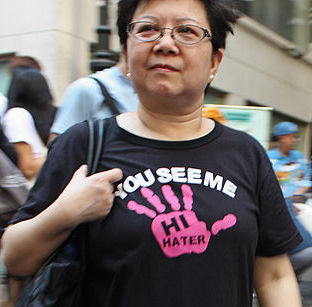# Hatred of Eleven IIWe define an "Eleven Hater" as a number that is not a multiple of eleven, but becomes a multiple of $11$ when any one digit is removed.

For clarity, consider a 3 digit number $\overline {abc}$. if $\overline {abc}$ is an "Eleven Hater", then $\overline {ab}, \overline{ac},$ and $\overline{bc}$ should all be multiples of $11$. Note that the order of the digits are not to be altered.

How many 5-digit Eleven Haters are there?

×